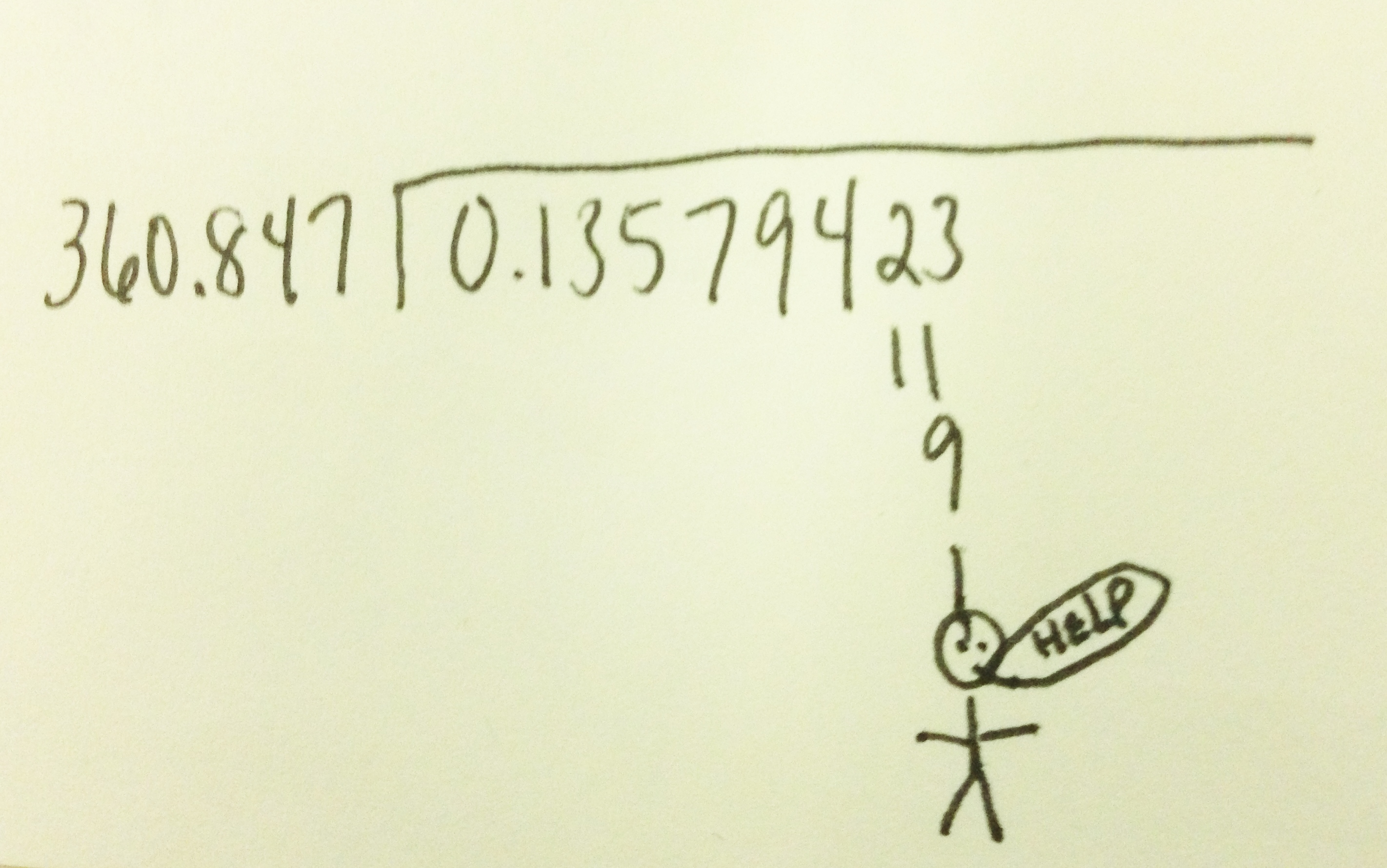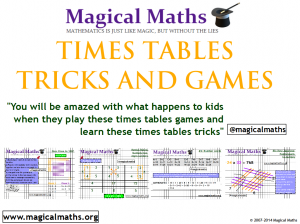Why did my Maths teacher not show me this? Mental Math Division tricks !!Math Division  – Mental Maths Tricks

Are you looking to develop your Math Division mental maths skills? If so, then you have come to the right place! Dividing integers can be tricky but the following rules will help you decide whether a number can be divisible by another single digit number. This is a great set of mental maths tricks to help you do this and one that should be shown to all budding mathematicians. Do not forget to take a look at another set of mental maths tricks about ways in which to learn your times tables.

Developing mental maths is very important in Mathematics and it is a skill that is gradually being neglected in schools across the globe. Learners are getting more and more dependent on technology and struggle to even do the most simple mental Maths.

Comments are FREE, please leave one below.Maths Division Tricks

Divisible by 2

• When a number is even i.e. the number ends in 0,2,4,6,8

Divisible by 3

• When the sum of the digits is a multiple of 3 i.e. 462, 4+6+2 = 12, 12 is in the 3 times table, 462 is divisible by 3.

Divisible by 4

• When a number’s last 2 digits are divisible by 4 i.e. 12,624, 24 is in the 4 times table.

Divisible by 5

• When a number ends in 0 or 5.

Divisible by 6

• When a number is divisible by 2 and 3.

Divisible by 8

• When the last 3 digits of a number is in the 8 times table. i.e. 7168 , 168 is divisible by 8

Divisible by 9

• When the sum of the digits of a number is in the 9 times table.
Divisible by 10
• When a number ends with 0.

If you enjoyed using the resource above then make sure to check out the games and tricks available to help develop, practice and learn times tables skills.5 comments on “Why did my Maths teacher not show me this? Mental Math Division tricks !!”
1.Ralph says:

A great website with invaluable resources. I must share this with the kiddies and my colleagues. Much appreciated. Regards.

PS. I finally invested in a tablet(thanks to twitter) and I cannot imagine a life without it from now on. 🙂

•magicalmaths says:

Thanks for that!

2.sue says:

Interested in looking at resources and teaching ideas for adults

3.Leila ghaddab says:

A better question might be, “Why didn’t the writers of my school curriculum make space in it to teach us about divisibility tests and why they work?” The rules are fun, but without the background information about why they work, they’re just a magic trick.